← back

# WTF JavaScript Quiz — explanations

If you haven’t done the quiz, I recommend to check it out before reading the answers 😛 Here’s a link: http://jsquiz.wtf/.

### Question 1: What is NaN?

`js`typeof NaN; // ❓❓❓``
`js`typeof NaN; // ❓❓❓``

Correct answer: `number`.

#### Explanation

NaN (Not a Number) belongs to numeric data types. It’s used for unrepresentable, undefined, or missing values. NaN was specified in IEEE 754 floating-point standard over 35 years ago. In the ECMAScript standard Numbers are actually represented as IEEE 754 floats.

This is how NaN looks in floating-point (32-bit) precision: s 111 1111 1xxx xxxx xxxx xxxx xxxx xxxx. The exponent of NaN is filled with 1s.

### Question 2: Is string a String?

`js`"wtf" instanceof String; // ❓❓❓``
`js`"wtf" instanceof String; // ❓❓❓``

Correct answer: `false`.

#### Explanation

In JavaScript everything is an object except for primitives: string, number, bigint, boolean, undefined, and symbol. The instanceof operator doesn’t work with primitives (works only with objects), so it returns false for a primitive value “wtf”. For primitives we’re using typeof.

### Question 3: Arrays comparison

`js`[] == ![]; // ❓❓❓``
`js`[] == ![]; // ❓❓❓``

Correct answer: `true`.

#### Explanation

Let’s take it step by step, starting with the right-hand side expression.

1. Empty array is truthy in JavaScript. In other words Boolean([]) is true.
2. The ! operator is defined for boolean values only, so when applied to nonboolean value, JavaScript will automatically coerce it into a boolean. As [] is truthy, ![] results in false.
3. Based on 1. and 2., we can replace the right-hand side expression with false, so we now need to consider: [] == false.
4. In this kind of comparison (object type and boolean type), JavaScript will convert values into numeric ones.(The Abstract Relational Comparison Algorithm)
5. [] is coerced to 0, and false is also coerced to 0, thus 0 == 0 is true.

🤯

### Question 4: Prototypes and global variables

`js`Object.prototype.wtf = "wtf";console.log(wtf); // ❓❓❓``
`js`Object.prototype.wtf = "wtf";console.log(wtf); // ❓❓❓``

Correct answer: “wtf” will be printed to the console.

#### Explanation

JavaScript is prototypal object oriented language. As window is an object, you can find Object.prototype in window’s prototype chain.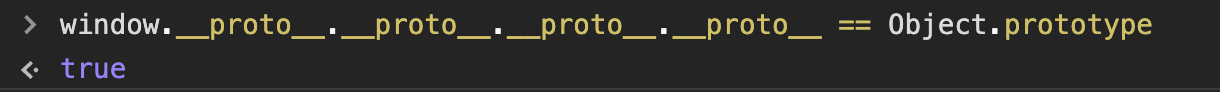In the code from the question, we set variable “wtf” to Object.prototype. In JavaScript, global variables are read from window but if window doesn’t have its own wtf property, JavaScript traverses window’s prototype chain to find wtf.

What’s more — now almost everything has “wtf” property 🙃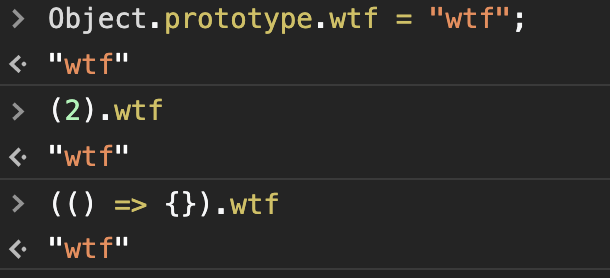### Question 5: Octal numbers

`js`const num = 02020;console.log(num); // ❓❓❓``
`js`const num = 02020;console.log(num); // ❓❓❓``

Correct answer: `1040`

#### Explanation

In JavaScript (as well as in other programming languages) octal (base-8) numbers start from 0.

2020 = (2 × 8³) + (0 × 8²) + (2 × 8¹) + (0 × 8⁰) = 1040

### Question 6: Min value

`js`Number.MIN_VALUE > 0; // ❓❓❓``
`js`Number.MIN_VALUE > 0; // ❓❓❓``

Correct answer: `true`.

#### Explanation

Number.MIN_VALUE is a very small, but a positive number — 5e-324.

### Question 7: Binary vs unary plus

`js`"foo" + +"bar"; // ❓❓❓``
`js`"foo" + +"bar"; // ❓❓❓``

Correct answer: `fooNaN`.

##### Explanation

As we have double +, the first one is a standard binary addition, but the second one is an unary plus.

In other words we can read the above code as: foo + (+“bar”).

The unary plus applied to “bar” will try to coerce it to number. However “bar” is not a number or numeric string, so it’s instead converted to NaN.

### Question 8: Number prototype

`js`(1) === 1; // trueNumber.prototype.isOne = (function () {  return this === 1;})(1).isOne(); // ❓❓❓``
`js`(1) === 1; // trueNumber.prototype.isOne = (function () {  return this === 1;})(1).isOne(); // ❓❓❓``

Correct answer: `false`.

#### Explanation

I don’t know 🙃

My guess would be that this is a boxed reference to a number, so what happens here is a comparison by reference.

The code below results in true, which possibly can mean that this needed to be coerced to number in order to perform a comparison by value.

`js`Number.prototype.isOne = function () { return this - 1 === 0; }(1).isOne();``
`js`Number.prototype.isOne = function () { return this - 1 === 0; }(1).isOne();``

### Question 9: arrrrays wtf

`js`++[[]][+[]]; // ❓❓❓``
`js`++[[]][+[]]; // ❓❓❓``

Correct answer: `1`.

#### Explanation

This one is well explained by John Resig on Hacker News.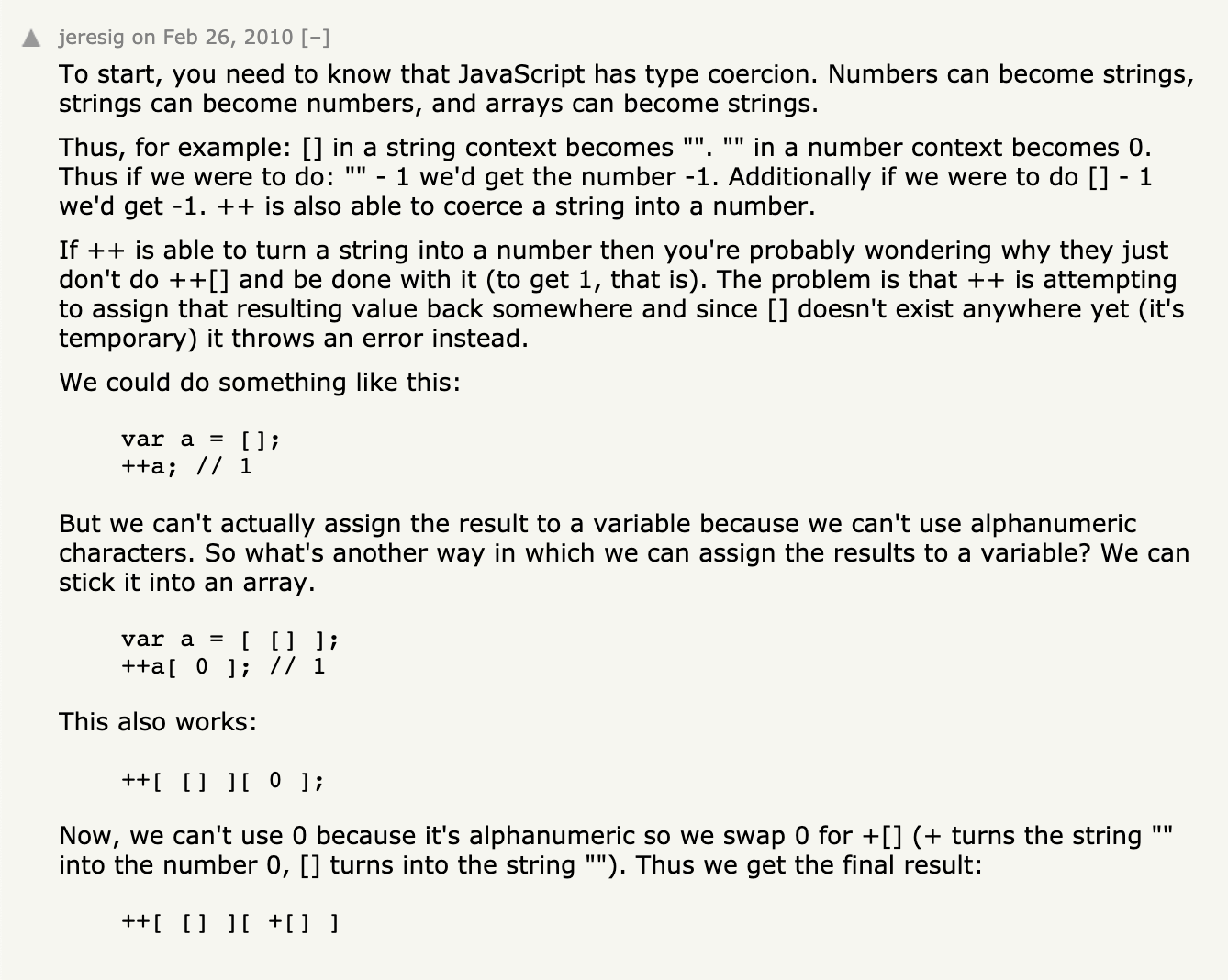### Question 10: constructor[constructor][constructor]

`js`const WTF = "constructor";WTF[WTF][WTF]("alert('wtf')")();``
`js`const WTF = "constructor";WTF[WTF][WTF]("alert('wtf')")();``

#### Explanation

Let’s break it into smaller pieces and substitute WTF with “constructor”.

1. WTF[WTF] becomes constructor[‘constructor’]. As window has its own constructor and global variables are read from window, we can easily access it. Now, the constructor of a constructor is a Function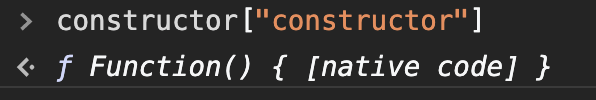1. Function[‘constructor’] is a Function. We could have one “WTF” less or five more if we wanted.
2. Function called with a string creates a function with the body being this string. And then, the trailing parentheses () call the function, evaluating “alert(‘wtf’)”.

Calling the constructor directly can create functions dynamically but suffers from security and similar (but far less significant) performance issues to eval. However, unlike eval, the Function constructor creates functions that execute in the global scope only.

### Question 11: Calling toString() on a number

`js`5.toString(); // Uncaught SyntaxError: Invalid or unexpected token5..toString(); // ❓❓❓``
`js`5.toString(); // Uncaught SyntaxError: Invalid or unexpected token5..toString(); // ❓❓❓``

Correct answer: `"5"`

#### Explanation

Firstly, why is 5.toString() a syntax error? It’s because JavaScript expects numbers followed by a dot to be floats.

5. itself is a valid floating point literal and it’s interpreted as 5.0. Thus executing 5.0.toString() works just fine.

####Question 12: parseInt in base-24

`js`parseInt(null, 24); // ❓❓❓``
`js`parseInt(null, 24); // ❓❓❓``

Correct answer: `23`

#### Explanation

The digits for base 24 are: 0, 1, 2, 3, 4, 5, 6, 7, 8, 9, a, b, c, d, e, f, …, n.

parseInt(null, 24) is equivalent to parseInt(“null”, 24), as JavaScript will convert the first argument to string.

Starting from the left-hand side of string “null”, the first digit n is 23rd digit in base-24. The next digit — u — is invalid (does not occur in base-24), so the rest of the string is ignored, and 23 is being returned.

### Question 13: Math.min vs Math.max

`js`Math.min() < Math.max(); // ❓❓❓``
`js`Math.min() < Math.max(); // ❓❓❓``

Correct answer: `false`

#### Explanation

`js`Math.max(); // -InfinityMath.min(); // Infinity``
`js`Math.max(); // -InfinityMath.min(); // Infinity``

Therefore Infinity < - Infinity results in false.

### Question 14: Parsing “Infinity” in base-24

`js`parseInt("Infinity", 24); // ❓❓❓``
`js`parseInt("Infinity", 24); // ❓❓❓``

Correct answer: `151176378`

#### Explanation

The digits for base-24 are: 0, 1, 2, 3, 4, 5, 6, 7, 8, 9, a, b, c, d, e, f, …, n.

Let’s look at Infinity’s letters as base-24 numbers:

`sh`i: 18n: 23f: 15i: 18n: 23i: 18t: invalid, does not occur in base-24y: ignored, as a previous digit was invalid``
`sh`i: 18n: 23f: 15i: 18n: 23i: 18t: invalid, does not occur in base-24y: ignored, as a previous digit was invalid``

Now we need to convert it with base-24:

151176378 = 18 * 240 + 23 * 241 + 15 * 242 + 18 * 243 + 23 * 244 + 18 * 245

`js`[18, 23, 18, 15, 23, 18].reduce((acc, n, i) => acc + n * 24 ** i, 0);> 151176378``
`js`[18, 23, 18, 15, 23, 18].reduce((acc, n, i) => acc + n * 24 ** i, 0);> 151176378``

### Question 15: Join on a sparse array

`js`[, , ,].join(); // ❓❓❓``
`js`[, , ,].join(); // ❓❓❓``

Correct answer: `",,"`

#### Explanation

[, , ,] is an array of length 3:Join by default uses a comma as a separator, and as we have a 3-elements array, the last comma is stripped as a trailing one, so the result is ”,,”.

### Question 16: Local storage

`js`localStorage = undefined;if (localStorage) {  console.log("wtf");}``
`js`localStorage = undefined;if (localStorage) {  console.log("wtf");}``

#### Explanation

Local storage only accepts string values, so when we set localStorage, to undefined, it’s converted to a string — “undefined”.

#### Question 17: document.all

`js`typeof document.all; // objecttypeof document.all; // ❓❓❓``
`js`typeof document.all; // objecttypeof document.all; // ❓❓❓``

Correct answer: `undefined`

#### Explanation

For this one, let’s look at the HTML spec.

The all attribute must return an HTMLAllCollection rooted at the Document node, whose filter matches all elements.

The object returned for all has several unusual behaviors:

1. The ToBoolean abstract operation in JavaScript returns false when given objects implementing the HTMLAllCollection interface.

2. The Abstract Equality Comparison algorithm, when given objects implementing the HTMLAllCollection interface, returns true when compared to the undefined and null values. (Comparisons using the Strict Equality Comparison algorithm, and Abstract Equality comparisons to other values such as strings or objects, are unaffected.)

3. The typeof operator in JavaScript returns the string “undefined” when applied to objects implementing the HTMLAllCollection interface.

The unusual behavior that we’re seeing in this example is the last one.

The rationale from the same spec:

These special behaviors are motivated by a desire for compatibility with two classes of legacy content: one that uses the presence of document.all as a way to detect legacy user agents, and one that only supports those legacy user agents and uses the document.all object without testing for its presence first.

### Question 18: Global regex

`js`const r = new RegExp("wtf", "gi");r.test("wtf"); // truer.test("wtf"); // ❓❓❓``
`js`const r = new RegExp("wtf", "gi");r.test("wtf"); // truer.test("wtf"); // ❓❓❓``

Correct answer: `false`

#### Explanation

Okay, so first things first.

There’s something like lastIndex:

The lastIndex is a read/write integer property of regular expression instances that specifies the index at which to start the next match.

When we’re using a global regex, methods test and exec will increase the lastIndex if the result is true (test) or not null (exec). Further calls of those functions will start searching the string from the lastIndex. Only when test returns false (or exec returns null), the lastIndex will be reset to 0.

This is what happens in the example:

1. r.test(“wtf”);lastIndex at first is 0, we found a substring matching the regex. lastIndex is updated to 3.
2. r.test(“wtf”);lastIndex is 3, and starting from the char of index 3, we get an empty string, so the result is false.

Note: As long as test() returns true, lastIndex will not reset—even when testing a different string!

### Question 19: Setting array properties

`js`const a = [1, 2, 3];a[-1] = "wtf";a[-1]; // ❓❓❓a[a.indexOf(-1)]; // ❓❓❓a[a.indexOf(100)]; // ❓❓❓``
`js`const a = [1, 2, 3];a[-1] = "wtf";a[-1]; // ❓❓❓a[a.indexOf(-1)]; // ❓❓❓a[a.indexOf(100)]; // ❓❓❓``

Correct answer: `"wtf", "wtf", "wtf"`

#### Explanation

There’s an algorithm for defining arrays’ properties.

Now, let’s consider these three cases to see what the algorithm does:

1. array = 0 — set first element of array to be 0.
Result: .

2. array[‘1’] = 1 — coerce ‘1’ to a number and set first element of array to be 1.
Result: [0, 1].

3. array[‘a’] = ‘a’“a” can’t be coarced to a number, so it will add key-value to the array.
Result: [0, 1, a: ‘a’].

With a[-1] = “wtf” we have a case number 3, because -1 is a negative number, thus can’t be used as an index in array a. The array after this operation will look like this: [1,2,3, -1: “wtf”].

a[-1] — we just retrive a value for key -1, so the result is “wtf”.

a[a.indexOf(-1)] and a[a.indexOf(100)]indexOf(x) returns position of the array with element x, and -1 if there’s no element x in the array. In our case there’s no -1 or 100 in array a, so we have just a[-1] and a[-1].

### Question 20: Array and object addition

`js`[] + {}; // ❓❓❓``
`js`[] + {}; // ❓❓❓``

Correct answer: `"[object Object]"`

#### Explanation

JavaScript converts both left-hand side and right-hand side values to strings. For an empty array it results in an empty string, whereas a string version of an object is “[object Object]“.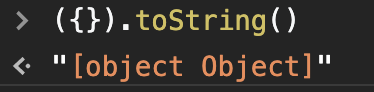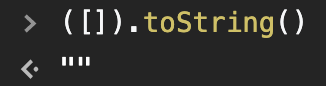Please correct me if I got something wrong!